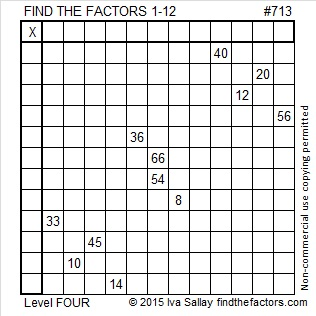# 713 and Level 4

• 713 is a composite number.
• Prime factorization: 713 = 23 x 31
• The exponents in the prime factorization are 1 and 1. Adding one to each and multiplying we get (1 + 1)(1 + 1) = 2 x 2 = 4. Therefore 713 has exactly 4 factors.
• Factors of 713: 1, 23, 31, 713
• Factor pairs: 713 = 1 x 713 or 23 x 31
• 713 has no square factors that allow its square root to be simplified. √713 ≈ 26.7020598.Go ahead and give this Level 4 puzzle a try:Print the puzzles or type the solution on this excel file: 12 Factors 2015-12-14

———————————————————————————

Would you like to know a little more about 713?

713 can be written as the sum of consecutive numbers several ways:

• 356 + 357 = 713; that’s two consecutive numbers.
• 20 + 21 + 22 + 23 + 24 + 25 + 26 + 27 + 28 + 29 + 30 + 31 + 32 + 33 + 34 + 35 + 36 + 37 + 38 + 39 + 40 + 41 + 42 = 713; that’s 23 consecutive numbers.
• 8 + 9 + 10 + 11 + 12 + 13 + 14 + 15 + 16 + 17 + 18 + 19 + 20 + 21 + 22 + 23 + 24 + 25 + 26 + 27 + 28 + 29 + 30 + 31 + 32 + 33 + 34 + 35 + 36 + 37 + 38 = 713; that’s 31 consecutive numbers.

713 is also the sum of three consecutive prime numbers: 233 + 239 + 241 = 713.

Two of its factors are 4 numbers away from their average, 27, so (23)(31) = (27 – 4)(27 + 4) = 27² – 4² = 713

713 is palindrome NN in BASE 30 (N = 23 in base 10); note that 23(30) +23(1) = 713.

———————————————————————————Thank you, Ricardo, for tweeting the puzzle and your work: